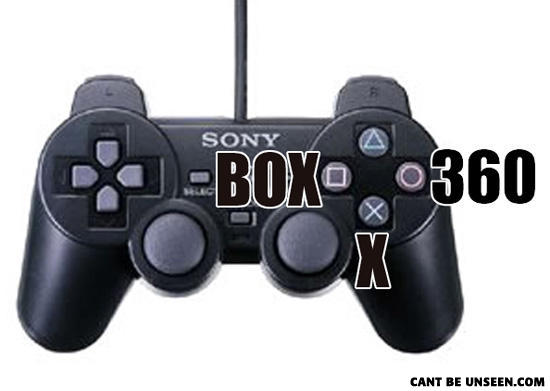1.1 by 2,079 huffers# Triangle =180, 360+180=540 Xbox 540?

## Comments On This Can't Be Unseen Picture

• Triangle =180, 360+180=540 Xbox 540?

• The "X BOX Then 360" form a triangle together with is the next button.

• my god! the triangle is a hint on what comes next!

• What is a Box X 360?

• triangle: red ring of death for retards who are colorblind

• So, if it is the Xbox 360, and the original was just the Xbox, then according to the Sony controller, where is the "X"